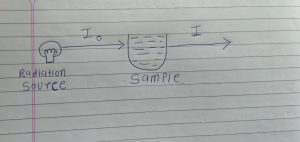# Absorption BASIC: Beer’s Law; Lambart’s Law and Equation

## Absorption BASIC: Beer’s Law; Lambart’s Law and Equation

Absorption BASIC

First we take one example we have one radio source and we have one sample.

And Incident radiation have 100% intensity. And it is denoted by Io.

When incident radiation is passed through out sample it is called transmitted radiation.

And it is denoted by I.Above condition we have two possible situation.

Situation 1. If sample solution is absorbed radiation

Absorption is more than 0.

Transparent is less than 100%.

So, Io>I

∴A=Io/I

∴T = I/Io

∴ A= log10 (1/T)

T =I/Io so, 1/T = Io/I

A= log10 ( Io/I)

Situation 2 :-If sample solution is transparent.

A% is 0, T% is 100%

∴ Io= I

Beer’s Law :-

When a beam of monochromatic radiation is passed through the absorbing radiation then the decrease in the intensity of the radiation will be directly proportional to the concentration of the solution.

Equation for beer law is

A =  ξ *C

Where,

A = absorption

ξ = molar extinction co-efficient

C = conc. of the solution

Lambart’s Law

When a beam of monochromatic radiation is passed through the absorbing medium then the decrease in the intensity of radiation will be directly proportional to the thickness of the solution.

Lamber’s Law Equation

A = ξ*L

Where

A = absorption

ξ = molar extinction co-efficient

L = path length

Beer – Lambert’s Law :-

When a beam of monochromatic radiation is passed through the absorbing medium then the decrease in the intensity of the radiation is directly proportional to the thickness of the medium and the concentration of the solution.

Equation :-

A = ξ*L*C

For More Standard and Quality Question Bank you can Join Our Test Series Programme for GPAT, NIPER JEE, Pharmacist Recruitment Exam, Drug Inspector Recruitment Exams, PhD Entrance Exam for Pharmacy